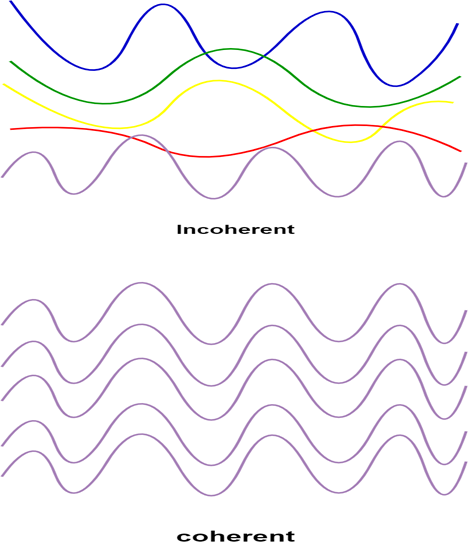Goseeko blog# What is Coherence?

## Definition:–

A predictable correlation of the amplitude and phase at any one point with another point is call as coherence. That means if two or more waves of same frequency are in same phase or have constant phase difference then these waves are coherent in nature.Figure: Incoherent and Coherent wave

In the case of conventional light, the property of coherence exhibits between a source and its virtual source. Whereas in the case of laser the property coherence exists between any two or more light waves.

## Types of coherence

There are two types of coherence

i) Temporal coherence

ii) Spatial coherence

## Temporal coherence (or Longitudinal coherence) :-

Temporal coherence is described as a direction of propagation having correlation of amplitude and phase at one point on the wave train w. r. t. another point on the same wave train. It is also call as longitudinal coherence.

Let us consider two points P1 and P2 on the same wave train, which is continuous as shown in the figure.

Figure : Wave train

If the value of phase and amplitude at any point is known, then we can easily determine the amplitude and phase for any other point on the same wave train by using the wave equation

y= a sin (ct-x)

where ‘x’ is the displacement of the wave at any instant of time ‘t’ and ‘a’ is the amplitude of the wave.

## Spatial coherence (or Transverse coherence):-

Spatial coherence describes correlation  in a plane perpendicular to the direction of propagation. It is defined as the predictable correlation of amplitude and phase at one point on the wave train w. r. t. another point on a second wave, then the waves are said to be spatial coherence. Spatial coherence is also call as transverse coherence.

Interested in learning about similar topics? Here are a few hand-picked blogs for you! *list*